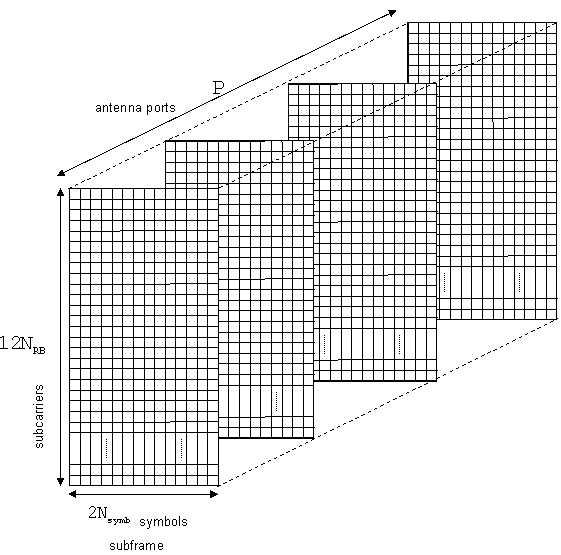Documentation

Representing Resource Grids

Overview

This section describes the data structures used to represent the resource grid in the LTE Toolbox™.

Multidimensional Arrays

Before OFDM modulation (IFFT), the physical channels and signals in LTE are mapped to different portions of the resource grid. LTE Toolbox represents the resource grid as a multidimensional array.The rows of this array represent the subcarrier. The columns map the OFDM or SC-FDMA symbols in the downlink and uplink, respectively. The third dimension or plane represents the antenna ports. In the LTE Toolbox, the resource grid spans a subframe in the time-domain, instead of a slot. Hence, the documentation uses the term subframe resource grid. The size of this multidimensional array is 12NRB×2Nsymb×P, where NRB is the number of resource blocks spanning the available bandwidth, Nsymb is the number of OFDM, or SC-FDMA in the uplink, symbols per slot, and P is the number of antenna ports. Therefore, the resource grid represents a subframe,two slots, and whole bandwidth since there are 12 subcarriers per resource block. For the single antenna case, you can work with a two-dimensional array of size 12NRB×2Nsymb.

Creating an Empty Resource Grid

Create an empty downlink resource grid using two different methods. Valid and equivalent subframe resource grids can be created using the lteDLResourceGrid function or the MATLAB® zeros function.

Initialize required parameters

Create the parameter structure for normal cyclic prefix, nine downlink resource blocks, and one transmit antenna. Also define seven symbols per slot for use in the zeros function only.

enb.CyclicPrefix = 'Normal';
enb.NDLRB = 9;
enb.CellRefP = 1;
noSymbolsSlot = 7;

Create two empty resource grids

Create an empty subframe resource grid, using each method.

resourceGrid1 = lteDLResourceGrid(enb);
resourceGrid2 = zeros(enb.NDLRB*12, noSymbolsSlot*2, enb.CellRefP);

Confirm the grids are equal

Compare the two grid variables for equality using the MATLAB isequal function.

isequal(resourceGrid1,resourceGrid2)
ans = logical
1

Both approaches generate the same result. Use either approach to create an empty downlink resource grid. Similarly, an empty uplink resource grid could be created using lteULResourceGrid function or the MATLAB zeros function.